/

### Performance Measurement Theory 3. Performance attribution

Next page

3.1          Portfolios will typically contain several sectors, in which case, given the same linear approximation as used above, the total fund and benchmark returns,and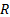will be as follows,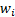= mean fund weighting for sector,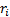= return for that individual sector etc,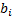= benchmark weighting for sectorand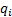= return on benchmark for sector: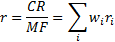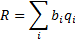where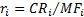and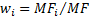3.2          Their difference is therefore as follows (since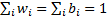):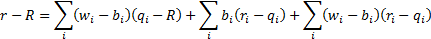3.3          We can rewrite the relative performance as follows: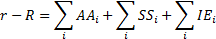where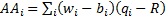,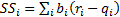and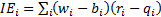.

3.4          The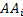are the contributions from ‘asset allocation’, the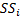are the contributions from ‘stock selection’ and the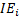are the contributions from an ‘interaction effect’. The interaction effect is the cross-product term that arises from the fact that the value added by stock selection is based on the amount of assets involved. Typically, the interaction effect is added into stock selection if you are a ‘top-down’ manager and into asset allocation if you are a ‘bottom-up’ manager.

3.5          The above analysis concentrates on additive attribution. To make the contributions from asset allocation and stock selection chain-link they can be restated in a geometric fashion as follows: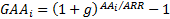and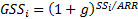(and a corresponding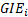if the interaction term is kept separate) where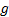= geometric relative return at total assets level,= additive relative return at total assets level andandare the additive asset allocation contribution and additive stock selection contribution from sector. Or, one can use natural logarithms using, say,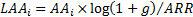so that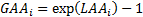. The total logarithmic contribution to return from a particular source over several periods can then be found merely by adding these terms together over.

3.6          Decomposing returns by ‘factors’ is conceptually quite similar. However, we also need:

(a)    For both fund and benchmark, the average exposure to each factor involved in the decomposition, say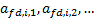and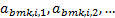(b)   For the benchmark only, the return a sector would deliver with zero factor exposure,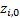and the extra return for a unit exposure to each individual factor (for each sector), say: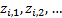so that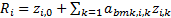.

3.7          The relative return can then be decomposed into:3.8          The first term is the contribution from asset allocation, the second the component of the stock selection explained by the various factors, and the third the residual component of stock selection which is unexplained by the various factors. The second term would normally be shown decomposed by both sector and factor. The sector analysis described above is a special case with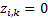and with more than one value for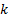. We would ideally want to build up the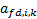by calculating the corresponding factor exposures by line of stock and then aggregating to the sector level. We might also do this for the benchmark as well or we might use a separate summarised data source.

3.9          Currency effects can be accommodated within this framework by including as separate ‘sectors’ any currency hedges away from the fund’s base position. If the base position is a hedged benchmark then these would be reverse hedges to reintroduce exposure to that currency. Performance measurers have developed lots of other ways of taking currency into account, although many only seem particularly relevant for certain ways in which currency decisions might be taken vis-à-vis sector or security selection decisions.